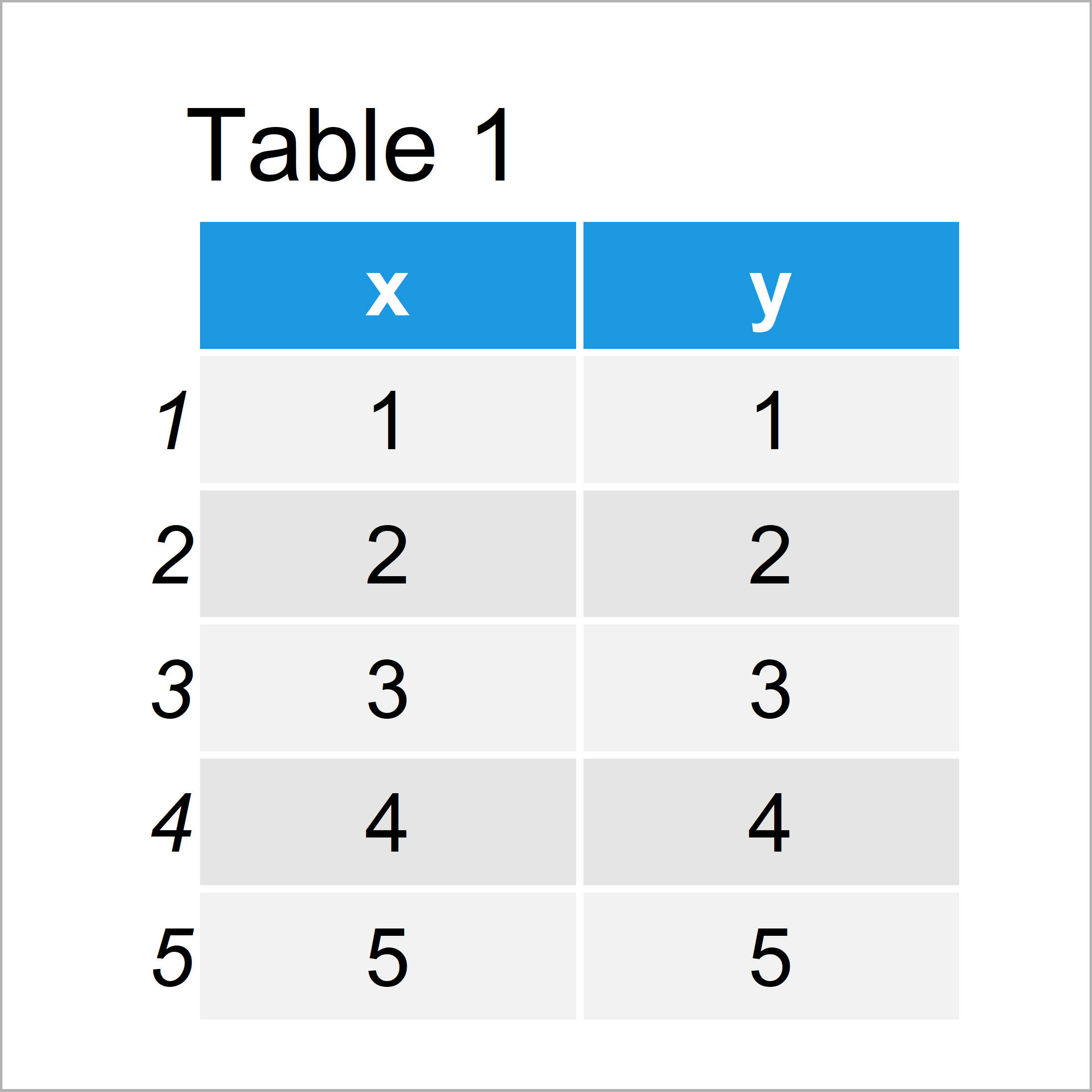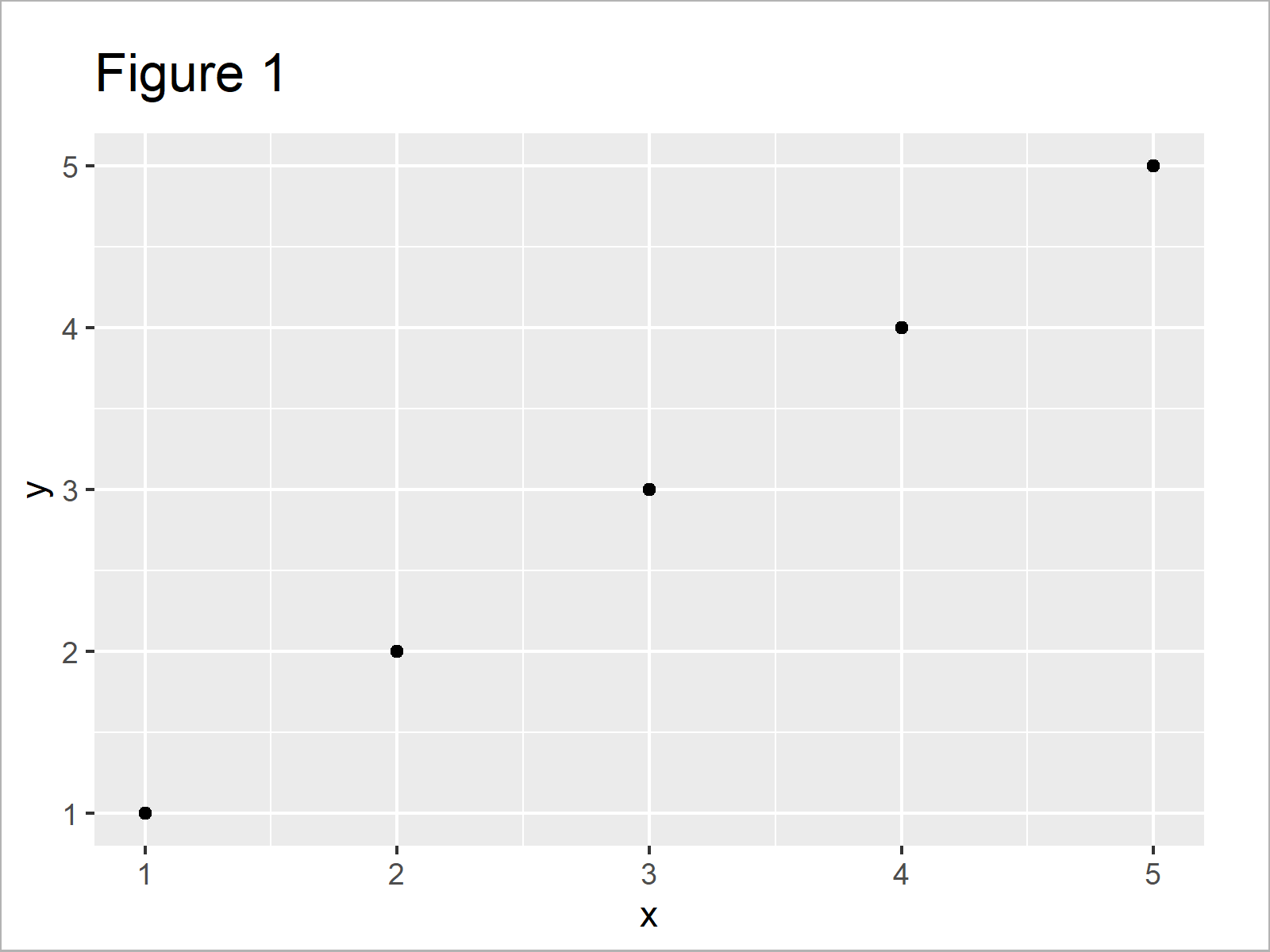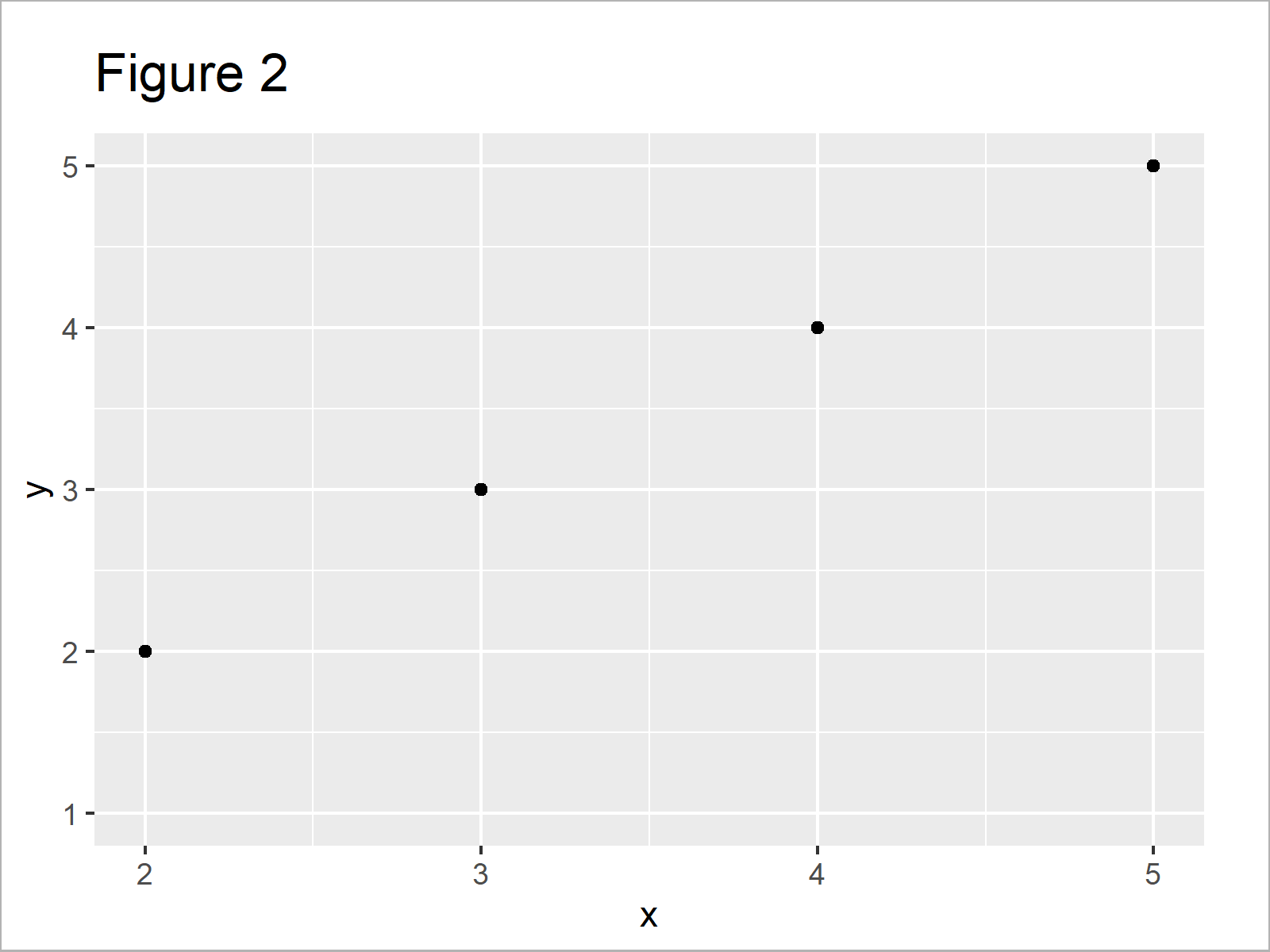# R ggplot2 Warning Message: Removed rows containing missing values

In this R article you’ll learn how to replicate and fix the ggplot2 warning message “Removed X rows containing missing values”.

The table of content looks as follows:

Let’s get started…

## Example Data, Packages & Basic Graph

Have a look at the following example data:

```data <- data.frame(x = 1:5, # Create example data y = 1:5) data # Print example data```As you can see based on Table 1, our example data is a data frame and comprises five rows and two numeric columns.

If we want to use the functions of the ggplot2 package, we also have to install and load ggplot2:

```install.packages("ggplot2") # Install ggplot2 package library("ggplot2") # Load ggplot2```

As next step, we can plot our data:

```ggp <- ggplot(data, aes(x, y)) + # Create ggplot2 with default axis limits geom_point() ggp # Draw ggplot2 plot```In Figure 1 it is shown that we have created a scatterplot showing five data points.

## Example: Reproduce the Warning Message – Removed X rows containing missing values

The following R programming code illustrates how to create the warning message “Removed X rows containing missing values”.

Let’s assume that we want to manually create axis limits in our ggplot2 graphic. Then, we can use the scale_x_continuous function as shown below:

```ggp + # Modify axis limits scale_x_continuous(limits = c(2, 5)) # Warning message: # Removed 1 rows containing missing values (geom_point).```After running the previous R syntax the scatterplot with narrower x-axis limits shown in Figure 2 has been created.

You can also see in the RStudio console that the warning message “Removed 1 rows containing missing values (geom_point).” has been returned.

The reason for that is that we have cut-off some data points from our plot by setting manual axis limits.

This is only a warning – So if you have intended to remove certain values from your plot this is not a problem.

However, when you see this warning message you should check whether any important data points have been removed.

I have recently published a tutorial that explains how to properly zoom into ggplot plots without removing any data. You can find it here.

## Video, Further Resources & Summary

In case you need further info on the R syntax of this article, you may want to have a look at the following video of my YouTube channel. In the video, I’m explaining the examples of this tutorial in a live session in RStudio:

Furthermore, you may want to have a look at some of the other articles of my website:

You have learned in this article how to deal with the warning “Removed X rows containing missing values” in the R programming language. Let me know in the comments section below, in case you have further questions.

Subscribe to the Statistics Globe Newsletter